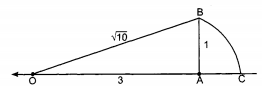# How to locate √x on number ...

How to locate √x on number line

Sia 🤖 1 year, 1 month ago

We can write 10 as
{tex}10 = 9 + 1 = 3^2 + 1^2{/tex}
Draw OA = 3 units, on the number line
Draw BA = 1 unit, perpendicular to OA.
Join OB
Figure:Now, by Pythagoras theorem,
{tex}OB^2 = AB^2 + OA^2\\ OB^2 = 1^2 + 3^2 = 10{/tex}
Taking O as centre and OB as a radius, draw an arc which intersects the number line at point C.
Clearly, OC corresponds to {tex}\sqrt{10}{/tex} on the number line.

Related Questions:## myCBSEguide

Trusted by 70 Lakh Students

#### CBSE Test Generator

Create papers in minutes

Print with your name & Logo

3 Lakhs+ Questions

Solutions Included

Based on CBSE Blueprint

Best fit for Schools & Tutors

#### Work from Home

• Work from home with us
• Create questions or review them from home

No software required, no contract to sign. Simply apply as teacher, take eligibility test and start working with us. Required desktop or laptop with internet connection

﻿